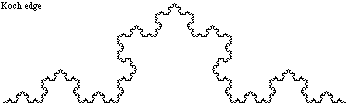index: click on a letter A B C D E F G H I J K L M N O P Q R S T U V W X Y Z A to Z index index: subject areas numbers & symbols sets, logic, proofs geometry algebra trigonometry advanced algebra & pre-calculus calculus advanced topics probability & statistics real world applications multimedia entrieswww.mathwords.com about mathwords website feedback

 Fractal A figure that is self-similar. That is, no matter how far you zoom in on the figure, the portion you look at is similar to the original figure. The Koch edge, below, is a fractal. Note: The word fractal is often used loosely to describe figures that do not quite meet this definition.